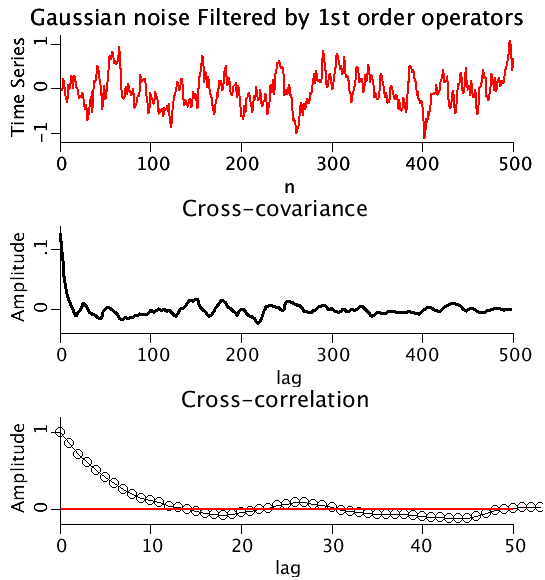# CrossCovariance

Model number
0356

Compute the crosscovariance for the output of two similar first order operators whose input is the same random variable.

## Description

```A random variable (Gaussianly distributed random numbers
with mean = 0 and variance = 1), RanVar, is filtered by
two different first order operators of the form

tau1*d(input1)/dt = RanVar-input1 and
tau2*d(input2)/dt = RanVar-input2.

The crosscovariance at a given lag is calculated

n
-----
\    input1(i)
Mean1 =   )   ---------
/     n
-----
i = 1

n
-----
\    input2(i)
Mean2 =   )   ---------
/     n
-----
i = 1

n - lag
-----
\
)    (input1(i) - Mean1) (input2(i + lag) - Mean2)
/
-----
i = 1
crosscovariance(lag) = ------------------------------------------------
n-1

The crosscovariance at zero lag  is the estimated covariance. The crosscorrelation
is the crosscovariance normalized by the product of the standard deviations
of each series..

crosscovariance(lag)
crossocorrelation(lag) = ---------------------
stdev1*stdev2```Upper Panel: The ouput of a 1st order operator with random Gaussian input.
Middle Panel: The cross-covariance as a function of lag.
Lower Panel: The cross-correlation (cross-covariance normalized by the product of the standard deviations).

## Equations

None.

The equations for this model may be viewed by running the JSim model applet and clicking on the Source tab at the bottom left of JSim's Run Time graphical user interface. The equations are written in JSim's Mathematical Modeling Language (MML). See the Introduction to MML and the MML Reference Manual. Additional documentation for MML can be found by using the search option at the Physiome home page.

References

None.

Key terms
crosscovariance
crosscorrelation
time series
1st order operator
lag
filter
xcovariance
xcorrelation
Acknowledgements

Please cite https://www.imagwiki.nibib.nih.gov/physiome in any publication for which this software is used and send one reprint to the address given below:
The National Simulation Resource, Director J. B. Bassingthwaighte, Department of Bioengineering, University of Washington, Seattle WA 98195-5061.

Model development and archiving support at https://www.imagwiki.nibib.nih.gov/physiome provided by the following grants: NIH U01HL122199 Analyzing the Cardiac Power Grid, 09/15/2015 - 05/31/2020, NIH/NIBIB BE08407 Software Integration, JSim and SBW 6/1/09-5/31/13; NIH/NHLBI T15 HL88516-01 Modeling for Heart, Lung and Blood: From Cell to Organ, 4/1/07-3/31/11; NSF BES-0506477 Adaptive Multi-Scale Model Simulation, 8/15/05-7/31/08; NIH/NHLBI R01 HL073598 Core 3: 3D Imaging and Computer Modeling of the Respiratory Tract, 9/1/04-8/31/09; as well as prior support from NIH/NCRR P41 RR01243 Simulation Resource in Circulatory Mass Transport and Exchange, 12/1/1980-11/30/01 and NIH/NIBIB R01 EB001973 JSim: A Simulation Analysis Platform, 3/1/02-2/28/07.